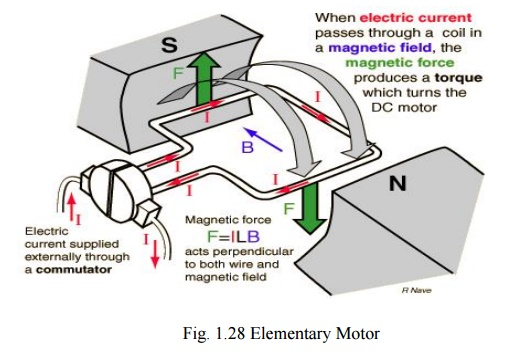Home | | Electrical Engineering and Instrumentation | Operating Principle of DC Motors and Torque Equation

# Operating Principle of DC Motors and Torque Equation

It is based on the principle that when a current-carrying conductor is placed in a magnetic field, it experiences a mechanical force whose direction is given by Fleming's Left-hand rule .

## Operating Principle of DC Motors

It is based on the principle that when a current-carrying conductor is placed in a magnetic field, it experiences a mechanical force whose direction is given by Fleming's Left-hand rule and whose magnitude is given by

Force, F = B I l Newton

Where, B is the magnetic field in weber/m2.

I is the current in amperes and

l is the length of the coil in meter.## 1. Torque Equation

Turning or twisting force about an axis is called torque.Consider a wheel of radius R meters acted upon by a circumferential force F Newton’s as shown in above figure. The wheel is rotating at a speed of N rpm.

The angular speed of the wheel ω = 2πN/60 rad/sec

Work done in one revolution W= Force x distanced travelled in one revolution

W = FX2πR joules

Power developed, P = Work done/time = W/Time for 1 rev.

P = FX2πR/(60/N) = (FXR)(2πN/60)

P = T x ω watts

Power in armature = armature torque x ω

EbIa = Ta x (2ΠN/60)

Where, Ta             = Armature torque.

Eb = PΦZN/60A

Substituting Eb values, we get,

Ta = 0.159ΦIa (PZ/A) N-m

Study Material, Lecturing Notes, Assignment, Reference, Wiki description explanation, brief detail
Electrical Engineering and Instrumentation : DC Machines : Operating Principle of DC Motors and Torque Equation |